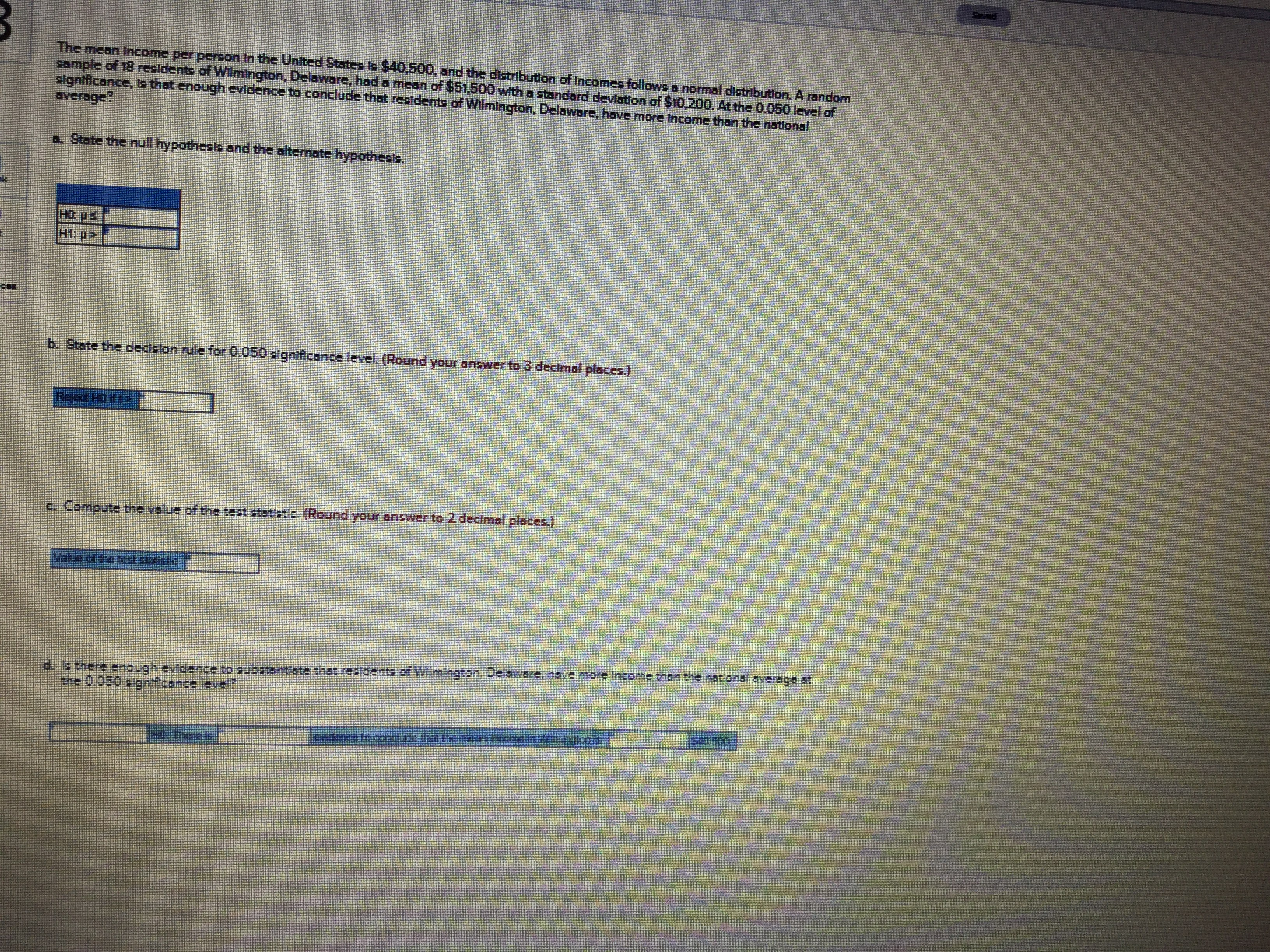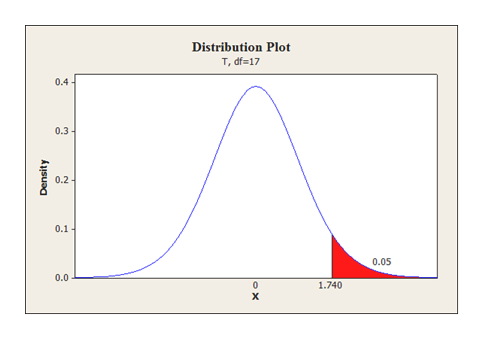# The mean Income per peron In the Unted States Is 40,500, and the distribution of Incomes follows a normal distribution. A randornsample of 18 resldents of Wilmington, Deaware, hod a rmean of \$51,500 with a standard deviation of \$10 200. At the 0.050 level ofsigntficance, E that enough cvidence to conclude that reoldents of Wilmington, Delawsrc, have more tncome than the national226euDADState the null hypothests and the alternate hypothests.H1 P?b. State the decislon rule for 0.050 elgntficance level. (Round your answer to 3 dectmal pleces.)ప్పయు 3c.Compute the value of the test stot'stic (Round your answer to 2 decimel places.)of Witmlngtan. Dabware. bave more Income than the nat onsl average atate thot r455u29no vBncuc c.ouaspthe 0.0s0 s gnfcance

Question
2 viewshelp_outlineImage TranscriptioncloseThe mean Income per peron In the Unted States Is 40,500, and the distribution of Incomes follows a normal distribution. A randorn sample of 18 resldents of Wilmington, Deaware, hod a rmean of \$51,500 with a standard deviation of \$10 200. At the 0.050 level of signtficance, E that enough cvidence to conclude that reoldents of Wilmington, Delawsrc, have more tncome than the national 226euDAD State the null hypothests and the alternate hypothests. H1 P? b. State the decislon rule for 0.050 elgntficance level. (Round your answer to 3 dectmal pleces.) ప్పయు 3 c.Compute the value of the test stot'stic (Round your answer to 2 decimel places.) of Witmlngtan. Dabware. bave more Income than the nat onsl average at ate thot r 455u29no vBncuc c.ouasp the 0.0s0 s gnfcance fullscreen
check_circle

Step 1

a.

The null and alternative hypothesis is obtained below:

Hypotheses:

Null hypothesis:

H0: µ ≤ 40,500

Alternative hypothesis:

H1: µ > 40,500

Since the alternative hypothesis states µ > 40,500, this test is a right-tailed test.

b.

The sample size (n) is 18

Degrees of freedom

Df = n – 1

= 18 –1

= 17

Step-by-step procedure to obtain the critical value using MINITAB software:

• Choose Graph > Probability Distribution Plot choose View Probability > OK.
• From Distribution, choose ‘t’ distribution.
• In Degrees of freedom, enter 17.
• Choose P Value and Right Tail for the region of the curve to shade.
• Enter the data value as 0.05.
• Click OK.

Output obtained using MINITAB software is given below:help_outlineImage TranscriptioncloseDistribution Plot T, df 17 0.4 0.3 0.2 0.1 0.05 0.0 0 1.740 Density ox fullscreen
Step 2

Here, the sample mean, x-bar is 51,500.

Population mean, µ is 40,500.

Sample standard deviation, s = 10,200

Sample size, n is 18.

The test statistic for t-test is calculated as 4.58 from the calculations given below.

Step 3

d.

Computation of P-value:

The right tailed P-value for the t-test is 0.000 which can be obtained using the excel formula, &ld...

### Want to see the full answer?

See Solution

#### Want to see this answer and more?

Solutions are written by subject experts who are available 24/7. Questions are typically answered within 1 hour.*

See Solution
*Response times may vary by subject and question.
Tagged in

### Other